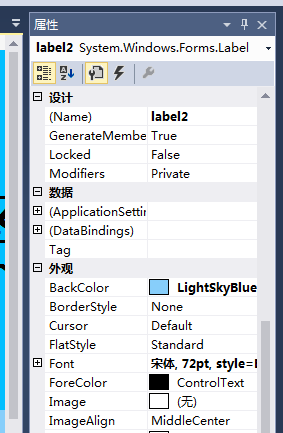c# 运用serialport与PLC串口通讯，读取（例如D200）的数据 80C

``````    private void btn_open_Click(object sender, EventArgs e)
{
int nSelect;
btn_open.Enabled = false;
Object selectedItem = cbxCOMPort.SelectedItem;
sp.PortName = selectedItem.ToString();//串口
selectedItem = cbxBaudRate.SelectedItem;
sp.BaudRate = int.Parse(selectedItem.ToString());//波特率
nSelect = cbxDataBits.SelectedIndex;//数据位
switch (nSelect)
{
case 0:
sp.DataBits = 8;
break;
case 1:
sp.DataBits = 7;
break;
case 2:
sp.DataBits = 6;
break;
case 3:
sp.DataBits = 5;
break;
}
nSelect = cbxParity.SelectedIndex;
switch (nSelect)
{
case 0:
sp.Parity = Parity.None;
break;
case 1:
sp.Parity = Parity.Odd;
break;
case 2:
sp.Parity = Parity.Even;
break;
case 3:
sp.Parity = Parity.Mark;
break;
case 4:
sp.Parity = Parity.Space;
break;
}
nSelect = cbxStopBits.SelectedIndex;
switch (nSelect)
{
case 0:
sp.StopBits = StopBits.None;
break;
case 1:
sp.StopBits = StopBits.One;
break;
case 2:
sp.StopBits = StopBits.OnePointFive;
break;
case 3:
sp.StopBits = StopBits.Two;
break;
}
sp.Open();
btn_close.Enabled = true;
}

private void btn_send_Click(object sender, EventArgs e)
{
textBox1.Text = "";
int n = 6;
byte[] buf = new byte[n];
string ee = textBox4.Text;
buf = Convert.ToByte(ee);
if (comboBox1.Text == "3X")
{
buf = Convert.ToByte(3);
}
else
{
buf = Convert.ToByte(4);
}
if (int.Parse(textBox5.Text) <= 255)
{
buf = Convert.ToByte(0);
buf = Convert.ToByte(int.Parse(textBox5.Text));
}
else
{
if (Convert.ToString(int.Parse(textBox5.Text), 16).Length < 4)
{
int b = Convert.ToInt32(Convert.ToString(int.Parse(textBox5.Text), 16).Substring(0, 1), 16);
buf = Convert.ToByte(b);
int c = Convert.ToInt32(Convert.ToString(int.Parse(textBox5.Text), 16).Substring(1, 2), 16);
buf = Convert.ToByte(c);
}
else
{
int b = Convert.ToInt32(Convert.ToString(int.Parse(textBox5.Text), 16).Substring(0, 2), 16);
buf = Convert.ToByte(b);
int c = Convert.ToInt32(Convert.ToString(int.Parse(textBox5.Text), 16).Substring(2, 2), 16);
buf = Convert.ToByte(c);
}
}

if (int.Parse(textBox6.Text) <= 255)
{
buf = Convert.ToByte(0);
buf = Convert.ToByte(int.Parse(textBox6.Text));
}
else
{
if (Convert.ToString(int.Parse(textBox6.Text), 16).Length < 4)
{
int d = Convert.ToInt32(Convert.ToString(int.Parse(textBox6.Text), 16).Substring(0, 1), 16);
buf = Convert.ToByte(d);
int f = Convert.ToInt32(Convert.ToString(int.Parse(textBox6.Text), 16).Substring(1, 2), 16);
buf = Convert.ToByte(f);
}
else
{
int d = Convert.ToInt32(Convert.ToString(int.Parse(textBox6.Text), 16).Substring(0, 2), 16);
buf = Convert.ToByte(d);
int f = Convert.ToInt32(Convert.ToString(int.Parse(textBox6.Text), 16).Substring(2, 2), 16);
buf = Convert.ToByte(f);
}
}
p = int.Parse(textBox6.Text) * 2 + 5;
byte[] buf1 = new byte[n + 2];
byte[] ReturnData = new byte;
ReturnData = CRC16_C(buf);
for (int i = 0; i < n; i++)
{
buf1[i] = buf[i];
}
buf1[n] = ReturnData;
buf1[n + 1] = ReturnData;
sp.Write(buf1.ToArray(), 0, n + 2);
}
{

byte[] TxData = new byte[n];//声明一个临时数组存储当前来的串口数据
m = m + n;
this.Invoke((EventHandler)
(delegate
{

Array.Resize(ref  TxData2, m);//改写数组大小
TxData.CopyTo(TxData2, m - TxData.Length);
if (m == p)
{
byte[] data = new byte[p - 2];
for (int i = 0; i < TxData2.Length - 2; i++)
{
data[i] = TxData2[i];
}
byte[] data2 = new byte;
data2 = TxData2[TxData2.Length - 2];
data2 = TxData2[TxData2.Length - 1];
byte[] data1 = new byte;
data1 = CRC16_C(data);
if (data1 == data2 && data1 == data2)
{
for (int j = 3; j <= m - 4; j = j + 2)
{
string s1 = TxData2[j].ToString();
string s2 = TxData2[j + 1].ToString();
string s = s1 + s2;
int g = Convert.ToInt32(s, 10);
string h = g.ToString();
textBox1.Text += h + "  ";
}
textBox7.Text = "读取成功";
}
else
{
textBox7.Text = "读取失败";
}
m = 0;
}

}
)
);
}
public byte[] CRC16_C(byte[] data)
{
byte CRC16Lo;
byte CRC16Hi;
byte CL; byte CH;
byte SaveHi; byte SaveLo;
byte[] tmpData;
int Flag;
CRC16Lo = 0xFF;
CRC16Hi = 0xFF;
CL = 0x01;
CH = 0xA0;
tmpData = data;
for (int i = 0; i < tmpData.Length; i++)
{
CRC16Lo = (byte)(CRC16Lo ^ tmpData[i]);
for (Flag = 0; Flag <= 7; Flag++)
{
SaveHi = CRC16Hi;
SaveLo = CRC16Lo;
CRC16Hi = (byte)(CRC16Hi >> 1);
CRC16Lo = (byte)(CRC16Lo >> 1);
if ((SaveHi & 0x01) == 0x01)
{
CRC16Lo = (byte)(CRC16Lo | 0x80);
}

if ((SaveLo & 0x01) == 0x01)
{
CRC16Hi = (byte)(CRC16Hi ^ CH);
CRC16Lo = (byte)(CRC16Lo ^ CL);
}
}
}
byte[] ReturnData = new byte;
ReturnData = CRC16Hi;
ReturnData = CRC16Lo;
return ReturnData;
``````

4个回答qq_42027587 回复qq_23126581: 所以能不能帮忙看看我这个下的程序对应的应该填什么，十分感谢qq_42027587 这我知道啊，界面是我自己搞的，就是不知道对应的对不对，而且我设备地址，初始地址，长度应该填什么啊，我发送了一直没反应，想知道发送的对不对，比如读取D200，我初始地址填200？那设备地址和长度填啥你点击那个控件然后看属性 最上面那行就是控件名，你把串口信息qq_42027587 这我知道啊，界面是我自己搞的，不知道对应的对不对，而且我设备地址，初始地址，长度应该填什么啊，发送了一直没反应

plc 都是 modbus协议 起始地址 00 00 长度 自定 去扫吧C#串口SerialPort总是收到数据
-
c#SerialPort接收数据的问题
-
c#SerialPort的数据处理问题
-
C#通过SerialPort读取电子天平数据时第一次操作无法获取数据，必须进行第二次操作才能获取当前数据？
-
c#中serialPort 连续发送3次，如果直接发送3次的话 会出现问题接收不完全或其他错误。
-
c#用serialport进行的串口数据接收，遇到了问题，求大神指点
-

-
C# serialPort 串口连接modem通信 64位系统不能通信
-
C# SerialPort 串口通信多线程问题
-

-
C#serialPort实现串口通信
-
C#中的serialport.Write与MSCOMM控件的put_output有什么区别？
-
C#与西门子200通讯，读取超过3个字节，界面就死机，求教
-
C#多个窗口使用用一个串口接受数据问题
-
【求助】c#SerialPort与Delphi的TApdComPort机制问题
-
C#串口数据读取问题求助
-
-
windows10系统中c#调用system.io.port SerialPort类不能通讯
-
c#编写的串口助手打开串口32单片机立刻黑屏，急求
-

996下的程序员，该如何保证自己的身体健康？

Spring高级技术梳理
Spring高级技术梳理 序言正文SpringDate部分Spring全家桶之SpringData——预科阶段Spring全家桶之SpringData——Spring 整合Hibernate与Hibernate JpaSpring全家桶之SpringData——Spring Data JPASpring全家桶之SpringData——SpringData RedisSpringBoot部分Sp...
Git 天天用 但是 Git 原理你了解吗？
Git 原理 做技术一定要知其然知其所以然，意思就是：知道它是这样的，更知道它为什么是这样的。我主要通过4块内容来简单介绍 Git 是原理是什么样的。这4块内容如下： Git 存储目录结构介绍 Git 是如何存储的 Git 的对象 Git引用 当然 Git 原理不仅仅包含这些，想要更深入了解请查看官方教程 https://git-scm.com/book/zh/v2/。 本文内容是我在 Git...
Android——微信自动回复实现

8000字干货：那些很厉害的人是怎么构建知识体系的

Java 网络爬虫，就是这么的简单

nginx学习，看这一篇就够了：下载、安装。使用：正向代理、反向代理、负载均衡。常用命令和配置文件

Java 爬虫遇上数据异步加载，试试这两种办法！

Angular 入门教程系列：39：使用ng-alain进行开发